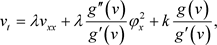# 一个非线性反应扩散方程的显式精确解Explicit Exact Solutions for a Nonlinear Reaction-Diffusion Equation

DOI: 10.12677/AAM.2020.91012, PDF, HTML, XML, 下载: 194  浏览: 330

Abstract: In this paper, a nonlinear reaction-diffusion equation is reduced to the standard heat equation by seeking appropriate nonlinear transformation and solves reduced equations with a variable separation approach, finally, through the inverse transformation of the nonlinear transformation, some new explicit exact solutions can be obtained.

1. 引言(1.1)

2. 相应的非线性变换

${u}_{t}=\lambda {u}_{xx}+ku,$ (2.1)

$u=g\left(v\right),$ (2.2)

${u}_{t}={g}^{\prime }\left(v\right){v}_{t},$ (2.3)

${u}_{xx}={g}^{″}\left(v\right){v}_{xx}+{g}^{\prime }\left(v\right){v}_{xx},$ (2.4)

${g}^{\prime }\left(v\right){v}_{t}=\lambda \left({g}^{″}\left(v\right){v}_{x}^{2}+{g}^{\prime }\left(v\right){v}_{xx}\right)+g\left(v\right),$ (2.5)(2.6)

$\left\{\begin{array}{l}{g}^{″}\left(v\right)/{g}^{\prime }\left(v\right)=\frac{2}{a-v}\\ {g}^{\prime }\left(v\right)/g\left(v\right)=\frac{1}{v}-\frac{1}{v-a}\end{array}$ (2.7)

$u=g\left(v\right)=\frac{v}{v-a},$ (2.8)

${\text{e}}^{-kt}{u}_{t}=\lambda {\text{e}}^{-kt}{u}_{xx}+k{\text{e}}^{-kt}u,$ (2.9)

$w={\text{e}}^{-kt}u,$ (2.10)

${w}_{t}=\lambda {w}_{xx}.$ (2.11)

$v=\frac{a{\text{e}}^{kt}w}{{\text{e}}^{kt}w\text{}-\text{}1},$ (2.12)

3. 显式精确解

$w\left(x,t\right)=X\left(x\right)T\left(t\right),$ (3.1)

${T}^{\prime }\left(t\right)X\left(x\right)=\lambda T\left(t\right){X}^{″}\left(x\right),$ (3.2)

$\frac{{T}^{\prime }\left(t\right)}{\lambda T\left(t\right)}=\frac{{X}^{″}\left(x\right)}{X\left(x\right)},$ (3.3)

${T}^{\prime }\left(t\right)=\lambda {\lambda }_{1}T\left(t\right),$ (3.4)

${X}^{″}\left(x\right)={\lambda }_{1}X\left(x\right).$ (3.5)

${\lambda }_{1}=0$ 时，通过求解方程(3.4)、(3.5)可得：

$T\left(t\right)=b,$ (3.6)

$X\left(x\right)=cx+d,$ (3.7)

${v}_{1}\left(x,t\right)=\frac{a{\text{e}}^{kt}\left({C}_{1}x+{C}_{2}\right)}{{\text{e}}^{kt}\left({C}_{1}x+{C}_{2}\right)-1},$ (3.8)

${\lambda }_{1}<0$ 时，求解常微分方程(3.4)、(3.5)可得通解：

$T\left(t\right)={a}_{1}{\text{e}}^{\lambda {\lambda }_{1}t},$ (3.9)

$X\left(x\right)={b}_{1}\mathrm{sin}\left(\sqrt{-{\lambda }_{1}}x\right)+{c}_{1}\mathrm{cos}\left(\sqrt{-{\lambda }_{1}}x\right),$ (3.10)

${v}_{2}\left(x,t\right)=\frac{a{\text{e}}^{\left(k+\lambda {\lambda }_{1}\right)t}\left({C}_{1}\mathrm{sin}\left(\sqrt{-{\lambda }_{1}}x\right)+{C}_{2}\mathrm{cos}\left(\sqrt{-{\lambda }_{1}}x\right)\right)}{{\text{e}}^{\left(k+\lambda {\lambda }_{1}\right)t}\left({C}_{1}\mathrm{sin}\left(\sqrt{-{\lambda }_{1}}x\right)+{C}_{2}\mathrm{cos}\left(\sqrt{-{\lambda }_{1}}x\right)\right)-1},$ (3.11)

${\lambda }_{1}>0$ 时，运用与上面相同的步骤可以求得如下显式精确解：

${v}_{3}\left(x,t\right)=\frac{a\left({C}_{1}{\text{e}}^{\left(k+\lambda {\lambda }_{1}\right)t+\sqrt{{\lambda }_{1}}x}+{C}_{2}{\text{e}}^{\left(k+\lambda {\lambda }_{1}\right)t-\sqrt{{\lambda }_{1}}x}\right)}{\left({C}_{1}{\text{e}}^{\left(k+\lambda {\lambda }_{1}\right)t+\sqrt{{\lambda }_{1}}x}+{C}_{2}{\text{e}}^{\left(k+\lambda {\lambda }_{1}\right)t-\sqrt{{\lambda }_{1}}x}\right)-1},$ (3.12)

$w\left(x,t\right)={C}_{1}{\text{e}}^{{k}_{1}x+\lambda {k}_{1}^{2}t+{\zeta }_{0}}+{C}_{2},$ (3.13)

$w\left(x,t\right)={\text{e}}^{-\lambda {k}_{1}^{2}t}\left({C}_{1}\mathrm{cos}\left({k}_{1}x+{\zeta }_{0}\right)+i{C}_{2}\mathrm{sin}\left({k}_{1}x+{\zeta }_{0}\right)\right)+{C}_{3},$ (3.14)

$w\left(x,t\right)={C}_{1}{x}^{2}+{C}_{2}x+2\lambda {C}_{1}t+{C}_{3},$ (3.15)

${v}_{4}\left(x,t\right)=\frac{a\left({C}_{1}{\text{e}}^{{k}_{1}x+\left(\lambda {k}_{1}^{2}+k\right)t+{\zeta }_{0}}+{C}_{2}{\text{e}}^{kt}\right)}{{C}_{1}{\text{e}}^{{k}_{1}x+\left(\lambda {k}_{1}^{2}+k\right)t+{\zeta }_{0}}+{C}_{2}{\text{e}}^{kt}-1},$ (3.16)

${v}_{5}\left(x,t\right)=\frac{a{\text{e}}^{\left(-\lambda {k}_{1}^{2}+k\right)t}\left({C}_{1}\mathrm{cos}\left({k}_{1}x+{\zeta }_{0}\right)+i{C}_{2}\mathrm{sin}\left({k}_{1}x+{\zeta }_{0}\right)\right)+a{C}_{3}{\text{e}}^{kt}}{{\text{e}}^{\left(-\lambda {k}_{1}^{2}+k\right)t}\left({C}_{1}\mathrm{cos}\left({k}_{1}x+{\zeta }_{0}\right)+i{C}_{2}\mathrm{sin}\left({k}_{1}x+{\zeta }_{0}\right)\right)+{C}_{3}{\text{e}}^{kt}-1},$ (3.17)

${v}_{6}\left(x,t\right)=\frac{a{\text{e}}^{kt}\left({C}_{1}{x}^{2}+{C}_{2}x+2\lambda {C}_{1}t+{C}_{3}\right)}{{\text{e}}^{kt}\left({C}_{1}{x}^{2}+{C}_{2}x+2\lambda {C}_{1}t+{C}_{3}\right)-1}.$ (3.18)

4. 结论与讨论

  Jimbo, M. and Miwa, T. (1983) Solitons and Infinite Dimensional Lie Algebras. Publications of the Research Institute for Mathematical Sciences, 19, 943-1001. https://doi.org/10.2977/prims/1195182017  Beal, R., Rabelo, M. and Tenenblat, K. (1988) Bäcklund Transformations and Inverse Scattering and for Some Pseudo-Spherical Surface Equations. Studies in Applied Mathematics, 81, 125-151. https://doi.org/10.1002/sapm1989812125  Wazwaz, A.M. (2013) On the noulocal Boussinesq Equation: Multiple-Soliton Solutions. Applied Mathematics Letters, 26, 1094-1098. https://doi.org/10.1016/j.aml.2013.06.008  Senthilvelan, M. (2001) On the Extended Applications of Homogeneous Balance Method. Applied Mathematics and Computation, 123, 381-388. https://doi.org/10.1016/S0096-3003(00)00076-X  Fan, E. and Zhang, H. (1998) A Note on the Homogeneous Balance Method. Physics Letters A, 246, 403-406. https://doi.org/10.1016/S0375-9601(98)00547-7  Kong, L.Q. and Dai, C.Q. (2015) Some Discussions about Variable Separations of Nonlinear Models Using Riccati Equation Expansion Method. Nonlinear Dynamics, 81, 1553-1561. https://doi.org/10.1007/s11071-015-2089-y  Zhang, S., Lou, S. and Qu, C. (2002) Variable Separation and Exact Solutions to Generalized Nonlinear Diffusion Equations. Chinese Physics Letters, 19, 1741-1744. https://doi.org/10.1088/0256-307X/19/12/301  Wazwaz, A.M. (2005) Exact Solutions to the Double Sinh-Gordon Equation by the Tanh Method and a Variable Separated ODE Method. Computers & Mathematics with Applications, 50, 499-508. https://doi.org/10.1016/j.camwa.2005.05.010  Wazwaz, A.M. (2004) A Sine-Cosine Method for Handling Nonlinear Wave Equation. Mathematical and Computer Modelling, 40, 499-508. https://doi.org/10.1016/j.mcm.2003.12.010  范恩贵, 张鸿庆. Whitham-Broer-Kaup浅水波方程的Bäcklund变换和精确解[J]. 应用数学与力学, 1998, 19(8): 667-670.  Shang, Y.D., Huang, Y. and Yuan, W.J. (2011) Bäcklund Transformations and Abundant Exact Explicit Solutions of the Sharma-Tasso-Olver Equation. Applied Mathematics and Computation, 217, 7172-7183. https://doi.org/10.1016/j.amc.2011.01.115  Grimson, M.J. and Barker, G.C. (1994) Continuum Model for the Spatiotemporal Growth of Bacterial Colonies. Physical Review E, 49, 1680-1684. https://doi.org/10.1103/PhysRevE.49.1680  Wang, D.S. and Zhang, Z.F. (2009) On the Integrability of the Generalized Fisher-Type Nonlinear Diffusion Equation. Journal of Physics A: Mathematical and Theoretical, 42, Article ID: 035209. https://doi.org/10.1088/1751-8113/42/3/035209  Wang, X.Y., Fan, S. and Kyu, T. (1997) Complete and Exact Solutions of a Class of Nonlinear Diffusion Equations and Problem of Velocity Selection. Physical Review E, 56, 4931-4934. https://doi.org/10.1103/PhysRevE.56.R4931  Bindu, P.S., Senthilvelan, M. and Lakshmanan, M. (2001) Singularity Structure, Symmetries and Integrability of Generalized Fisher Type Nonlinear Diffusion Equation. Journal of Physics A: Mathematical and General, 34, 689-696. https://doi.org/10.1088/0305-4470/34/49/101  Feng, Z.S. (2008) Traveling Wave Behavior for a Generalized Fisher Equation. Chaos, Solitons and Fractals, 38, 481-488. https://doi.org/10.1016/j.chaos.2006.11.031